# The FASTCLUS Procedure

### PROC FASTCLUS Statement

PROC FASTCLUS <MAXCLUSTERS=n> <RADIUS=t> <options> ;

The PROC FASTCLUS statement invokes the FASTCLUS procedure. You must specify the MAXCLUSTERS= option or RADIUS= option or both in the PROC FASTCLUS statement.

MAXCLUSTERS=n
MAXC=n

specifies the maximum number of clusters permitted. If you omit the MAXCLUSTERS= option, a value of 100 is assumed.

R=t

establishes the minimum distance criterion for selecting new seeds. No observation is considered as a new seed unless its minimum distance to previous seeds exceeds the value given by the RADIUS= option. The default value is 0. If you specify the REPLACE=RANDOM option, the RADIUS= option is ignored.

You can specify the following options in the PROC FASTCLUS statement. Table 36.1 summarizes the options available in the PROC FASTCLUS statement.

Table 36.1: PROC FASTCLUS Statement Options

Option

Description

Specify input and output data sets

Specifies input data set

Specifies input SAS data set previously created by the OUTSTAT= option

Specifies input SAS data set for selecting initial cluster seeds

Specifies divisor for variances

Output Data Processing

Specifies name for cluster membership variable in OUTSEED= and OUT= data sets

Specifies label for cluster membership variable in OUTSEED= and OUT= data sets

Specifies output SAS data set containing original data and cluster assignments

Specifies writing to OUTSEED= data set on every iteration

Specifies output SAS data set containing cluster centers

Specifies output SAS data set containing statistics

Initial Clusters

Permits cluster to seeds to drift during initialization

Specifies maximum number of clusters

Specifies minimum distance for selecting new seeds

Specifies seed to initializes pseudo-random number generator

Specifies seed replacement method

Clustering Methods

Specifies convergence criterion

Deletes cluster seeds with few observations

Optimizes an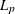criterion, where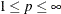Specifies maximum number of iterations

Prevents an observation from being assigned to a cluster if its distance to the nearest cluster seed is large

Arcane Algorithmic Options

Specifies number of bins used for computing medians for LEAST=1

Specifies criterion for updating the homotopy parameter

Specifies initial value of the homotopy parameter

Uses an iteratively reweighted least squares method instead of the modified Ekblom-Newton method for 1 < p < 2

Missing Values

Imputes missing values after final cluster assignment

Excludes observations with missing values

Control Displayed Output

Displays distances between cluster centers

Displays cluster assignments for all observations

Suppresses displayed output

Suppresses display of large matrices

Suppresses display of all results except for the cluster summary

Suppresses warning in output

The following list provides details on these options. The list is in alphabetical order.

BINS=n

specifies the number of bins used in the bin-sort algorithm for computing medians for LEAST=1. By default, PROC FASTCLUS uses from 10 to 100 bins, depending on the amount of memory available. Larger values use more memory and make each iteration somewhat slower, but they can reduce the number of iterations. Smaller values have the opposite effect. The minimum value of n is 5.

CLUSTER=name

specifies a name for the variable in the OUTSEED= and OUT= data sets that indicates cluster membership. The default name for this variable is CLUSTER.

CLUSTERLABEL=name

specifies a label for the variable CLUSTER in the OUTSEED= and OUT= data sets. By default this variable has no label.

CONVERGE=c
CONV=c

specifies the convergence criterion. Any nonnegative value is permitted. The default value is 0.0001 for all values of p if LEAST=p is explicitly specified; otherwise, the default value is 0.02. Iterations stop when the maximum relative change in the cluster seeds is less than or equal to the convergence criterion and additional conditions on the homotopy parameter, if any, are satisfied (see the HP= option). The relative change in a cluster seed is the distance between the old seed and the new seed divided by a scaling factor. If you do not specify the LEAST= option, the scaling factor is the minimum distance between the initial seeds. If you specify the LEAST= option, the scaling factor is an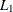scale estimate and is recomputed on each iteration. Specify the CONVERGE= option only if you specify a MAXITER= value greater than 1.

DATA=SAS-data-set

specifies the input data set containing observations to be clustered. If you omit the DATA= option, the most recently created SAS data set is used. The data must be coordinates, not distances, similarities, or correlations.

DELETE=n

deletes cluster seeds to which n or fewer observations are assigned. Deletion occurs after processing for the DRIFT option is completed and after each iteration specified by the MAXITER= option. Cluster seeds are not deleted after the final assignment of observations to clusters, so in rare cases a final cluster might not have more than n members. The DELETE= option is ineffective if you specify MAXITER=0 and do not specify the DRIFT option. By default, no cluster seeds are deleted.

DISTANCE  |  DIST

computes distances between the cluster means.

DRIFT

executes the second of the four steps described in the section Background. After initial seed selection, each observation is assigned to the cluster with the nearest seed. After an observation is processed, the seed of the cluster to which it is assigned is recalculated as the mean of the observations currently assigned to the cluster. Thus, the cluster seeds drift about rather than remaining fixed for the duration of the pass.

HC=c
HP=p<p>

pertains to the homotopy parameter for LEAST=p, where 1 < p < 2. You should specify these options only if you encounter convergence problems when you use the default values.

For 1 < p < 2, PROC FASTCLUS tries to optimize a perturbed variant of theclustering criterion (Gonin and Money; 1989, pp. 5–6).

When the homotopy parameter is 0, the optimization criterion is equivalent to the clustering criterion. For a large homotopy parameter, the optimization criterion approaches the least squares criterion and is therefore easy to optimize. Beginning with a large homotopy parameter, PROC FASTCLUS gradually decreases it by a factor in the range [0.01,0.5] over the course of the iterations. When both the homotopy parameter and the convergence measure are sufficiently small, the optimization process is declared to have converged.

If the initial homotopy parameter is too large or if it is decreased too slowly, the optimization can require many iterations. If the initial homotopy parameter is too small or if it is decreased too quickly, convergence to a local optimum is likely. The following list gives details on setting the homotopy parameter.

HC=c

specifies the criterion for updating the homotopy parameter. The homotopy parameter is updated when the maximum relative change in the cluster seeds is less than or equal to c. The default is the minimum of 0.01 and 100 times the value of the CONVERGE= option.

HP=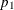specifiesas the initial value of the homotopy parameter. The default is 0.05 if the modified Ekblom-Newton method is used; otherwise, it is 0.25.

HP=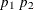also specifies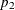as the minimum value for the homotopy parameter, which must be reached for convergence. The default is the minimum ofand 0.01 times the value of the CONVERGE= option.

IMPUTE

requests imputation of missing values after the final assignment of observations to clusters. If an observation that is assigned (or would have been assigned) to a cluster has a missing value for variables used in the cluster analysis, the missing value is replaced by the corresponding value in the cluster seed to which the observation is assigned (or would have been assigned). If the observation cannot be assigned to a cluster, missing value replacement depends on whether or not the NOMISS option is specified. If NOMISS is not specified, missing values are replaced by the mean of all observations in the DATA= data set having a value for that variable. If NOMISS is specified, missing values are replace by the mean of only observations used in the analysis. (A weighted mean is used if a variable is specified in the WEIGHT statement.) For information about cluster assignment see the section OUT= Data Set. If you specify the IMPUTE option, the imputed values are not used in computing cluster statistics.

If you also request an OUT= data set, it contains the imputed values.

INSTAT=SAS-data-set

reads a SAS data set previously created with the FASTCLUS procedure by using the OUTSTAT= option. If you specify the INSTAT= option, no clustering iterations are performed and no output is displayed. Only cluster assignment and imputation are performed as an OUT= data set is created.

IRLS

causes PROC FASTCLUS to use an iteratively reweighted least squares method instead of the modified Ekblom-Newton method. If you specify the IRLS option, you must also specify LEAST=p, where 1 < p < 2. Use the IRLS option only if you encounter convergence problems with the default method.

LEAST=p | MAX
L=p | MAX

causes PROC FASTCLUS to optimize ancriterion, where(Spath; 1985, pp. 62–63). Infinity is indicated by LEAST=MAX. The value of this clustering criterion is displayed in the iteration history.

If you do not specify the LEAST= option, PROC FASTCLUS uses the least squares (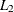) criterion. However, the default number of iterations is only 1 if you omit the LEAST= option, so the optimization of the criterion is generally not completed. If you specify the LEAST= option, the maximum number of iterations is increased to permit the optimization process a chance to converge. See the MAXITER= n option for details.

Specifying the LEAST= option also changes the default convergence criterion from 0.02 to 0.0001. See the CONVERGE= c for details.

When LEAST=2, PROC FASTCLUS tries to minimize the root mean squared difference between the data and the corresponding cluster means.

When LEAST=1, PROC FASTCLUS tries to minimize the mean absolute difference between the data and the corresponding cluster medians.

When LEAST=MAX, PROC FASTCLUS tries to minimize the maximum absolute difference between the data and the corresponding cluster midranges.

For general values of p, PROC FASTCLUS tries to minimize the pth root of the mean of the pth powers of the absolute differences between the data and the corresponding cluster seeds.

The divisor in the clustering criterion is either the number of nonmissing data used in the analysis or, if there is a WEIGHT statement, the sum of the weights corresponding to all the nonmissing data used in the analysis (that is, an observation with n nonmissing data contributes n times the observation weight to the divisor). The divisor is not adjusted for degrees of freedom.

The method for updating cluster seeds during iteration depends on the LEAST= option, as follows (Gonin and Money, 1989).

LEAST=p

Algorithm for Computing Cluster Seeds

p = 1

bin sort for median

1 < p < 2

modified Merle-Spath if you specify IRLS;

otherwise modified Ekblom-Newton

p = 2

arithmetic mean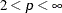Newton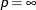midrange

During the final pass, a modified Merle-Spath step is taken to compute the cluster centers for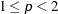or.

If you specify the LEAST=p option with a value other than 2, PROC FASTCLUS computes pooled scale estimates analogous to the root mean squared standard deviation but based on pth power deviations instead of squared deviations.

LEAST=p

Scale Estimate

p = 1

mean absolute deviation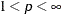root mean pth-power absolute deviationmaximum absolute deviation

The divisors for computing the mean absolute deviation or the root mean pth-power absolute deviation are adjusted for degrees of freedom just like the divisors for computing standard deviations. This adjustment can be suppressed by the VARDEF= option.

LIST

lists all observations, giving the value of the ID variable (if any), the number of the cluster to which the observation is assigned, and the distance between the observation and the final cluster seed.

MAXITER=n

specifies the maximum number of iterations for recomputing cluster seeds. When the value of the MAXITER= option is greater than zero, PROC FASTCLUS executes the third of the four steps described in the section Background. In each iteration, each observation is assigned to the nearest seed, and the seeds are recomputed as the means of the clusters.

The default value of the MAXITER= option depends on the LEAST=p option.

LEAST=p

MAXITER=

not specified

1

p = 1

20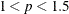50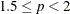20

p = 2

10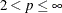20

MEAN=SAS-data-set

creates an output data set to contain the cluster means and other statistics for each cluster. If you want to create a SAS data set in a permanent library, you must specify a two-level name. For more information about permanent libraries and SAS data sets, see SAS Language Reference: Concepts.

NOMISS

excludes observations with missing values from the analysis. However, if you also specify the IMPUTE option, observations with missing values are included in the final cluster assignments.

NOPRINT

suppresses the display of all output. Note that this option temporarily disables the Output Delivery System (ODS). For more information, see Chapter 20: Using the Output Delivery System.

OUT=SAS-data-set

creates an output data set to contain all the original data, plus the new variables CLUSTER and DISTANCE. If you want to create a SAS data set in a permanent library, you must specify a two-level name. For more information about permanent libraries and SAS data sets, see SAS Language Reference: Concepts.

OUTITER

outputs information from the iteration history to the OUTSEED= data set, including the cluster seeds at each iteration.

OUTSEED=SAS-data-set
OUTS=SAS-data-set

is another name for the MEAN= data set, provided because the data set can contain location estimates other than means. The MEAN= option is still accepted.

OUTSTAT=SAS-data-set

creates an output data set to contain various statistics, especially those not included in the OUTSEED= data set. Unlike the OUTSEED= data set, the OUTSTAT= data set is not suitable for use as a SEED= data set in a subsequent PROC FASTCLUS step.

RANDOM=n

specifies a positive integer as a starting value for the pseudo-random number generator for use with REPLACE=RANDOM. If you do not specify the RANDOM= option, the time of day is used to initialize the pseudo-random number sequence.

REPLACE=FULL | PART | NONE | RANDOM

specifies how seed replacement is performed, as follows:

FULL

requests default seed replacement as described in the section Background.

PART

requests seed replacement only when the distance between the observation and the closest seed is greater than the minimum distance between seeds.

NONE

suppresses seed replacement.

RANDOM

selects a simple pseudo-random sample of complete observations as initial cluster seeds.

SEED=SAS-data-set

specifies an input data set from which initial cluster seeds are to be selected. If you do not specify the SEED= option, initial seeds are selected from the DATA= data set. The SEED= data set must contain the same variables that are used in the data analysis.

SHORT

suppresses the display of the initial cluster seeds, cluster means, and standard deviations.

STRICT
STRICT=s

prevents an observation from being assigned to a cluster if its distance to the nearest cluster seed exceeds the value of the STRICT= option. If you specify the STRICT option without a numeric value, you must also specify the RADIUS= option, and its value is used instead. In the OUT= data set, observations that are not assigned due to the STRICT= option are given a negative cluster number, the absolute value of which indicates the cluster with the nearest seed.

SUMMARY

suppresses the display of the initial cluster seeds, statistics for variables, cluster means, and standard deviations.

VARDEF=DF | N | WDF | WEIGHT | WGT

specifies the divisor to be used in the calculation of variances and covariances. The default value is VARDEF=DF. The possible values of the VARDEF= option and associated divisors are as follows.

Value

Description

Divisor

DF

error degrees of freedomN

number of observations

n

WDF

sum of weights DF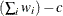WEIGHT | WGT

sum of weights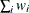In the preceding definitions, c represents the number of clusters.

VARIABLESAREUNCORRELATED

suppresses the warning, displayed in the listing when there are two or more VAR variables, concerning the validity of the Approximate Expected Over-All R-Squared and the Cubic Clustering Criterion when the variables used in clustering are correlated. Note that the FASTCLUS procedure does not compute correlations; the warning is for a potential problem.